# 阿森河铸铁气动隔膜泵的特点及技术参数名称 口径 中间体材质 过流部件材质 膜片材质 阀球材质 阀座材质 气动隔膜泵 0.6=1/4” 10=3/8”15=1/2” 20=3/4”25=1” 40=1.5” 50=2” 80=3” 100=4” PP=聚丙烯 AL=铝合金 AL=铝合金 PP=聚丙烯 PM=聚甲醛 KV=聚偏氟乙烯 TF=特氟龙 SS=不锈钢304 LL=不锈钢316 AC=铸铁 TF=特氟龙 ST=山道橡胶 HY=聚醚橡胶 VT=氟橡胶 BN=丁倩橡胶 TF=特氟龙 ST=山道橡胶 HY=聚醚橡胶 VT=氟橡胶 BN=丁倩橡胶 SS=不锈钢 TF=特氟龙 ST=山道橡胶 HY=聚醚橡胶 VT=氟橡胶 BN=丁倩橡胶 SS=不锈钢## 阿森河环保资讯展示

### 新闻动态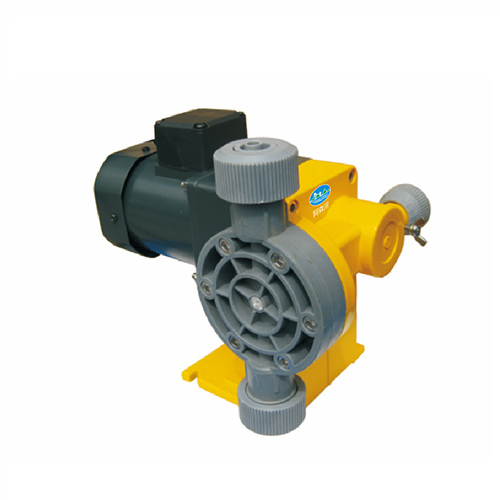#### 卧式计量泵(1)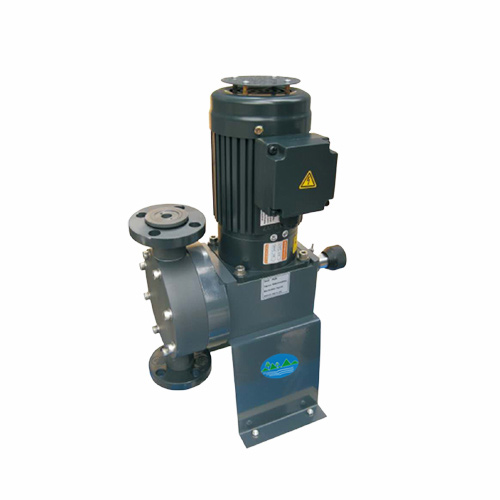#### 立式计量泵(1)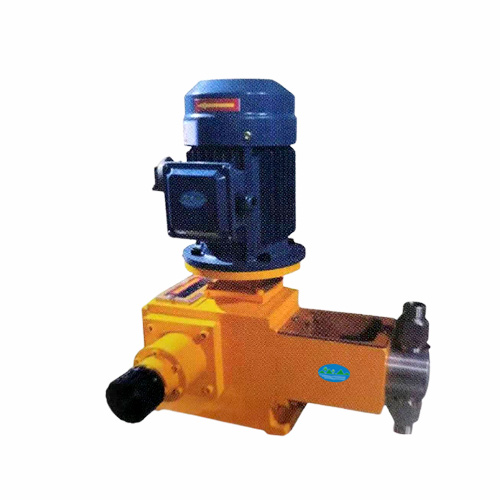#### 柱塞计量泵(1)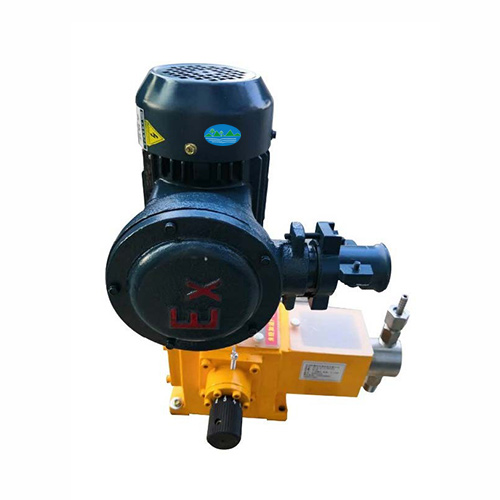#### 柱塞计量泵(2)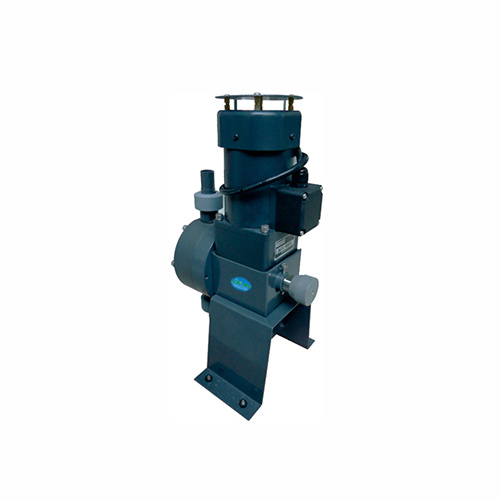#### 立式计量泵(2)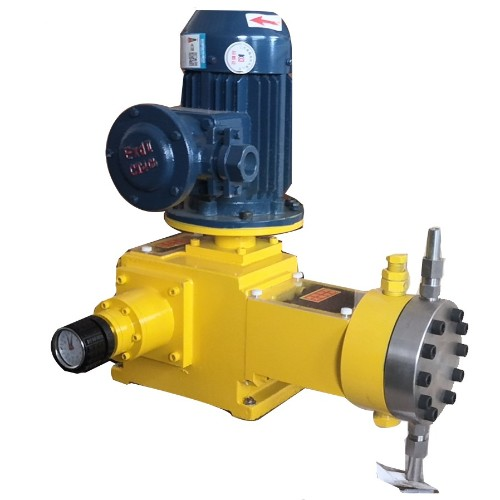#### 液压计量泵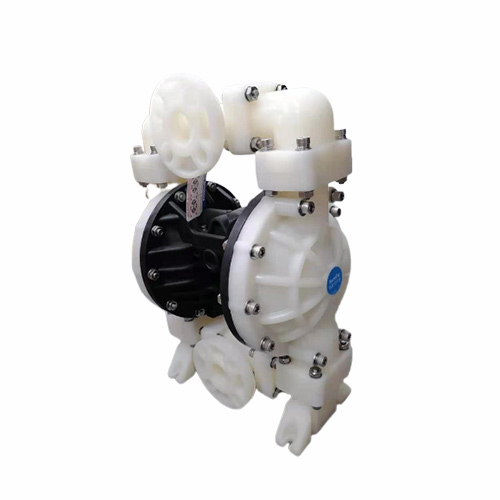#### 气动隔膜泵(1)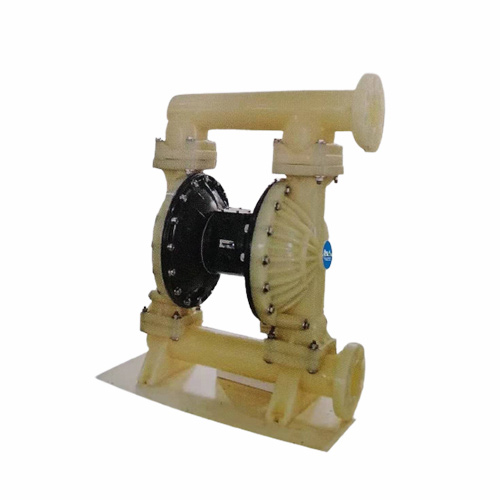#### 气动隔膜泵(2)#### 气动隔膜泵(3)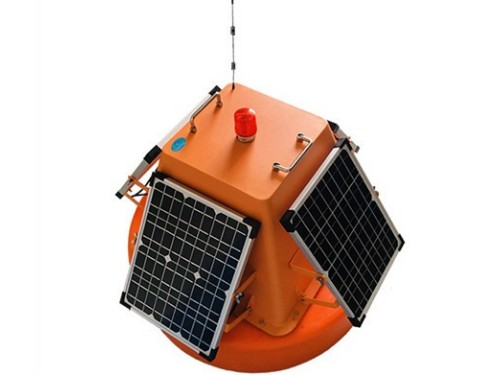#### 水质监测浮标（ASH-600）

18501539867
0512-80196586
•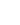Address

苏州市相城区元和街道如元路1688号东区111

•E-mail

396593188@qq.com

•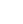Tel 18501539867 / 0512-80196586

##微信客服

• 服务热线

18501539867
0512-80196586
• 微信扫一扫